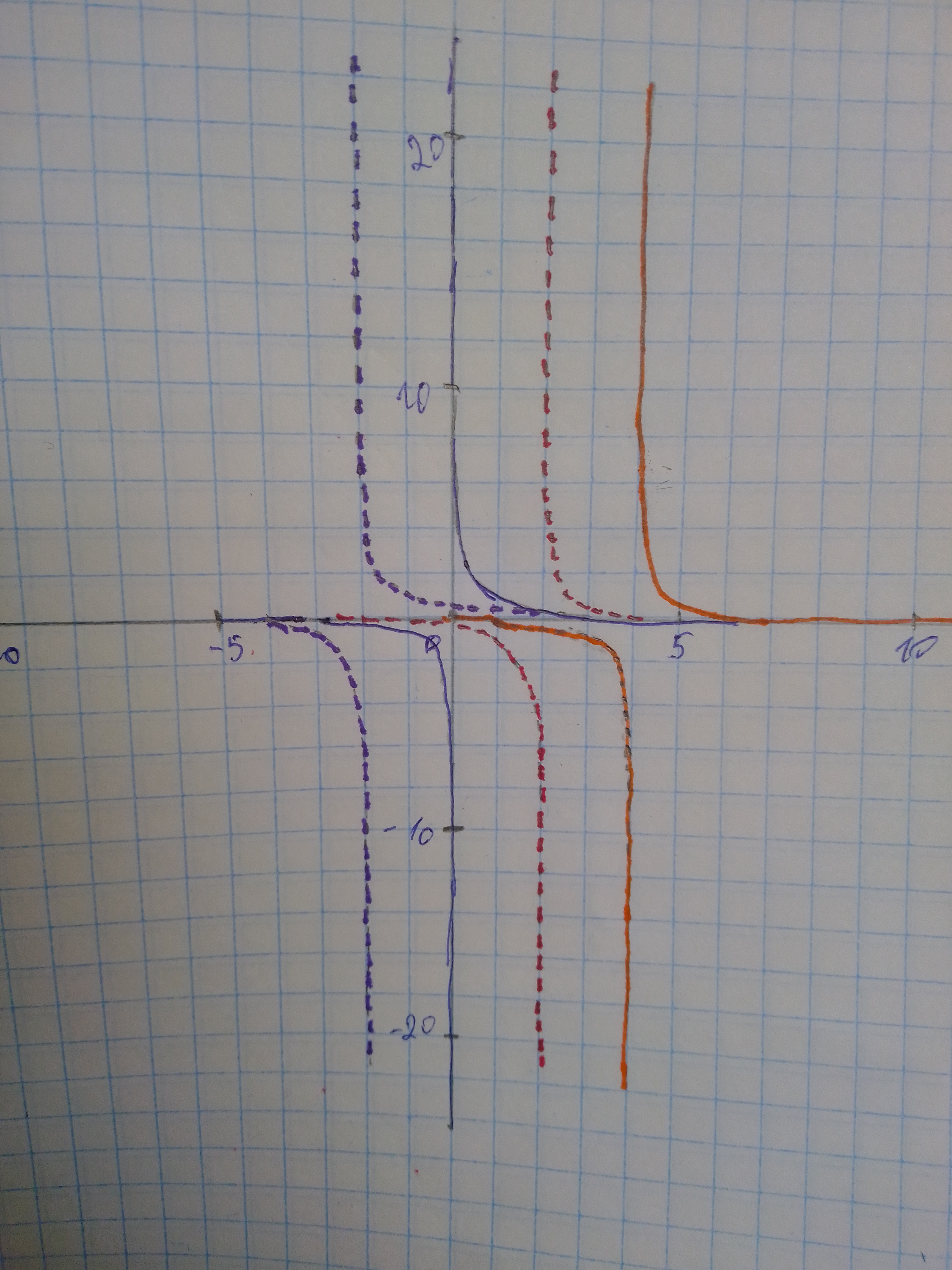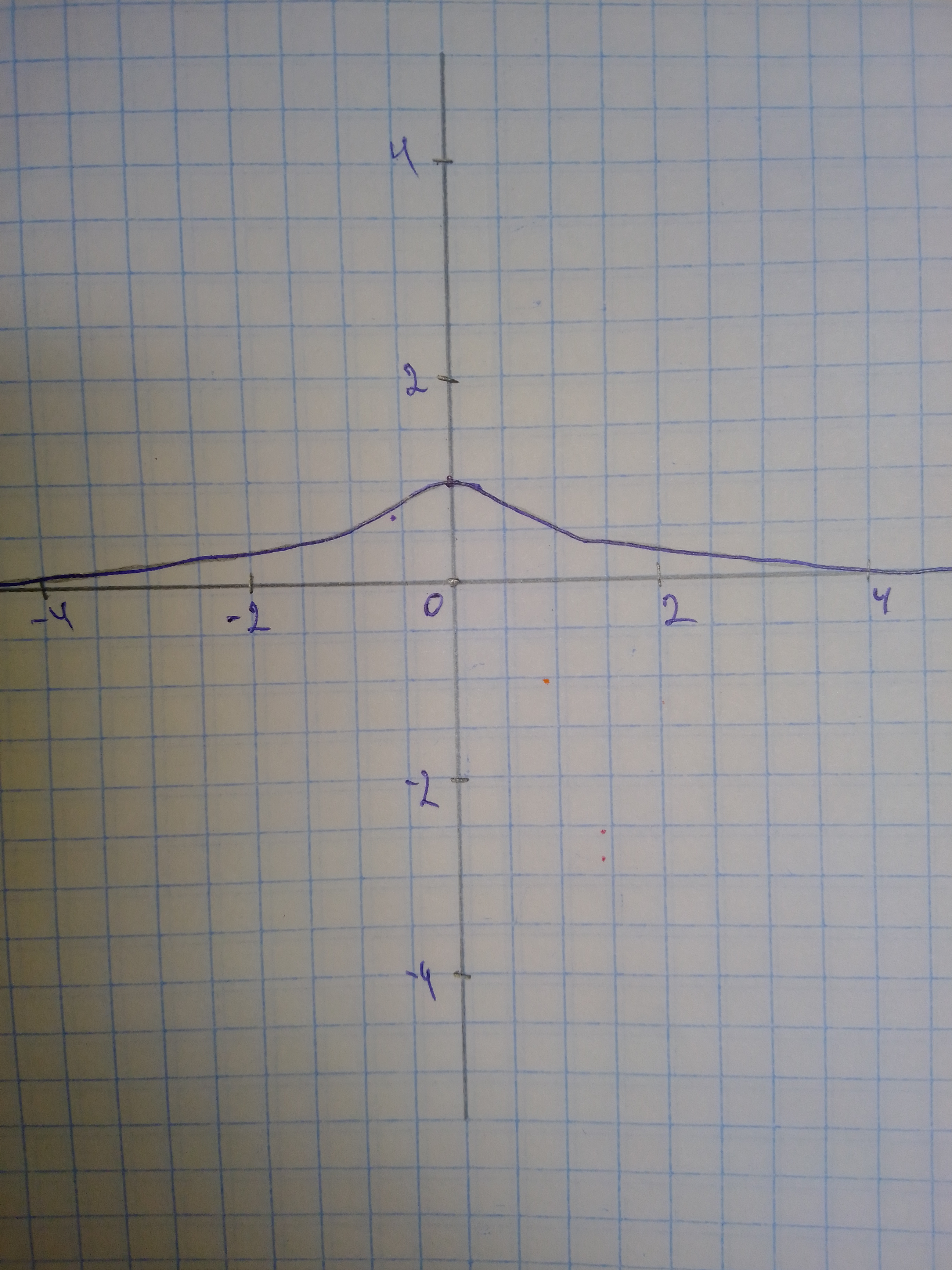# In the following items, you will analyze how several transformations affect the graph of the function f(x)=frac{1}{x}. Investigate the graphs of f(x)=avissidep 2020-12-15 Answered
In the following items, you will analyze how several transformations affect the graph of the function $f\left(x\right)=\frac{1}{x}$. Investigate the graphs of . If you use a graphing calculator, select a viewing window $±23.5$ for x and $±15.5$ for y. At what values in the domain did vertical asymptotes occur for each of the functions? Explain why the vertical asymptotes occur at these values.
You can still ask an expert for help

• Questions are typically answered in as fast as 30 minutes

Solve your problem for the price of one coffee

• Math expert for every subject
• Pay only if we can solve itViktor Wiley

Step 1 We graph f(x), as well as g(x) (dotted line), h(x) (dashed line), and p(x) (solid line).Step 2 We graph z(x):Step 3 We find that the vertical asymptotes are $f\left(x\right):x=0$
$g\left(x\right):x=-2$
$h\left(x\right):x=2$
$p\left(x\right):x=4$
$z\left(x\right):$ None Each vertical asymptote is found by finding the value(s) of x for which the denominator equals zero, and therefore the function is undefined. Note that there is no such real value of x in the case of z(x).